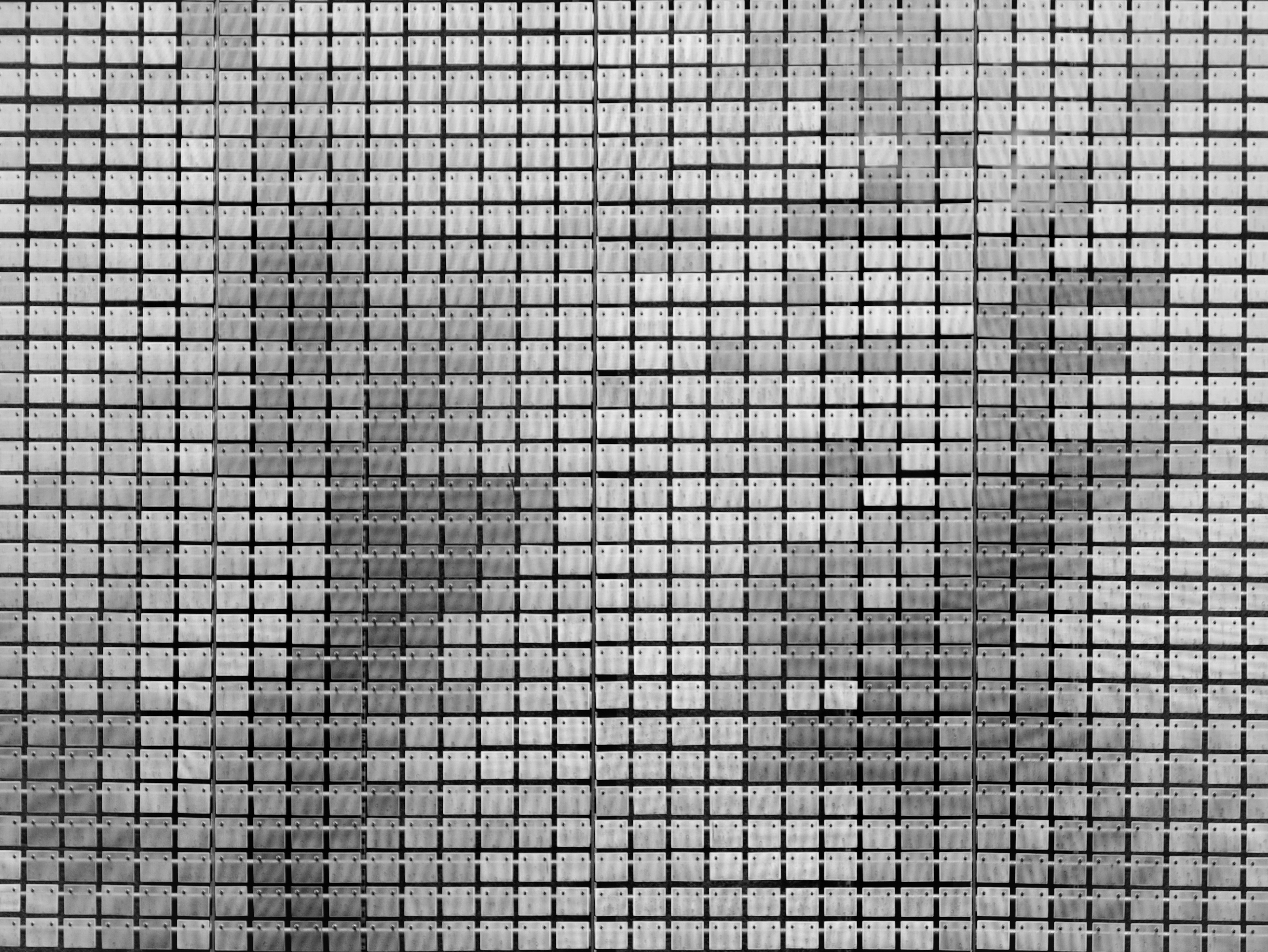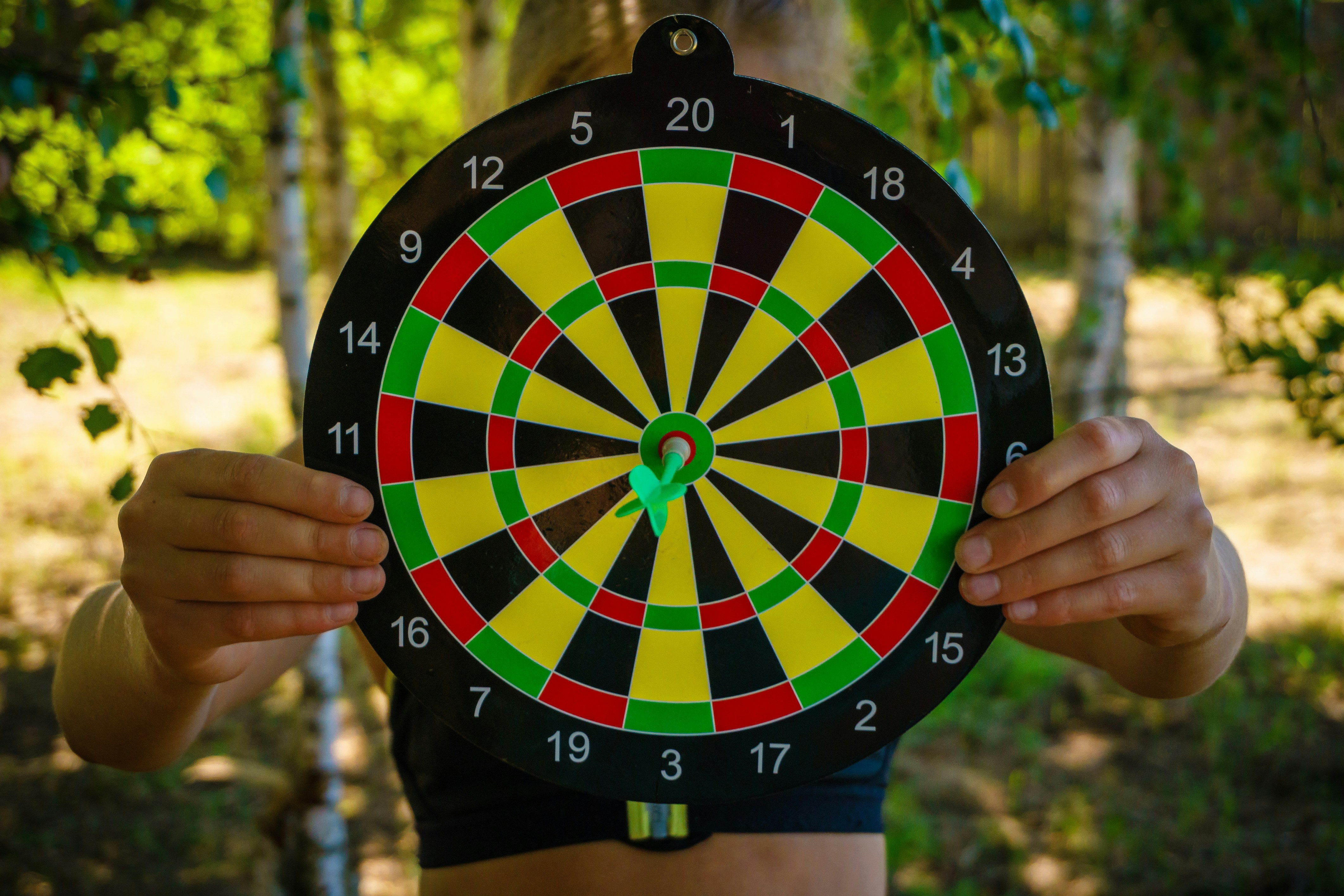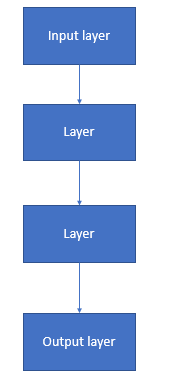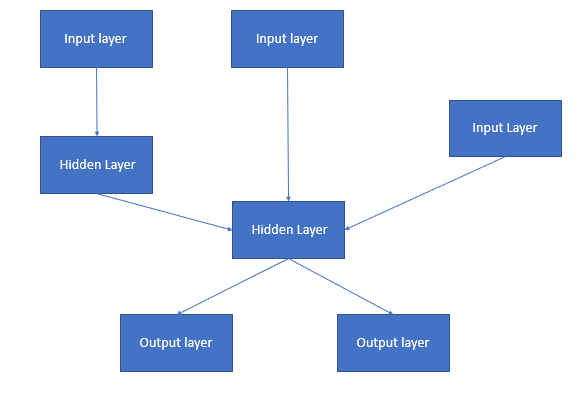# Keras Input Shape: The Beginning of Every Model

Creating different machine learning models in Keras becomes super easy once we understand the fundamentals.

Getting the correct output shape starts with correctly defining the right input shape for your deep learning models.

If you mess this up, you’ll spend a ton of time googling around to figure out why your model will not run correctly.

## What is the Keras Input Shape?

The Keras input shape is a parameter for the input layer (InputLayer). You’ll use the input shape parameter to define a tensor for the first layer in your neural network. If your input is an array of n integers, then your input shape would be (n,).

### Different Usages of the Input layer

When defining your input layer, you need to consider the specific Keras model you are building.

#### Image input shape

If your input data is an image and your model is a classification model, you’ll want to define the input shape by the number of pixels and channels.For classification models, think about your dataset being constrained to some subset of values; for example, if you’re trying to predict on the MNIST dataset (Source), your classification model will try to put each image into a group between [0,9]

A 250×250 pixel image with three channels will be

input_shape=(250,250,3)

However, if the model you are building is more regression-focused, your shape will be much different.

#### Array input_shape

Let’s say your input will be an array of 600 values; this means you’ll need to define your input shape a bit differently.

You will usually see an array of inputs in supervised learning, where you’re trying to find patterns in a dataset that lead you to a specific target column that you will predict (regression).

A 600-value array would look something like this.

input_shape=(600,)

Many people will try not to include the comma in the input_shape, but this comma is mandatory in Python.This is because tensors are created from tuples, and without the comma, Python does not transform this into a tuple, making it impossible for the Input function to create the tuple. (Read More)

##### Keras Input Shapes Batch Dimension

One of the most confusing aspects of the input shape when using Keras is understanding how batching works with this input tensor.

Along with batching, we get a ton of questions about how to know steps per epoch in Keras and we go over it in-depth in that linked article.

Since we’re defining only one instance of the training data, we may see None for the first dimension whenever we access our model during the training process.

In this Keras example

input_shape=(600,)

If you were to print this out in model.summary(), you would see this.

(None, 600)

This shape tuple responds with None in the first parameter due to the batch size.

Remember, each array was 600 values long, but during training, we will probably be passing in batches that have a similar structure.

If we were to pass in batches that have size 30, when checking our model.summary(), what we would see is

(30,600)

as we now have 30 tensors of 600 values (batch size).

Knowing how many dimensions is crucial for accurate modeling, and correctly orchestrating the next layer from the previous layer is how you create accurate models.Some people will try defining the batch size in their models; however, this can prove problematic.

Allowing Keras to choose the batch size without user contributions will allow for a fluid input size, meaning the batch size can change at any time.

This is optimal and will allow flexibility in your sequential model and output shape.

### Keras Sequential Model

In Keras, much of your modeling can be done with the Sequential parameter.

Some inputs you may need for this modeling tutorial

from tensorflow import keras

from tensorflow.keras import layers

Think of the sequential model as a one-way road, where the entrance will be your input layer, then go through some hidden layers to a single output layer.The input tensor is fundamental; remember, we can define that in a couple of different ways.

In newer versions of TensorFlow (Keras integrated), you’ll see the layer input defined as the following, using Conv2D (dense layers require inputs also).

CNNModel.add(layers.Conv2D(32, (3, 3), activation=’elu’, input_shape=(32, 32, 3)))

In older versions, you’ll see the Input layer defined as we discussed earlier with something like

Both of these will work the same way and have the same shape.

When using the model summary, you will be able to see the outline of the model.

If you do not define an input layer while defining your model, you will not be able to call the model summary method until your input shape is defined.

Using the model summary is one of the easiest ways to understand how your model will progress, as you’ll be able to see how the dense layers will be laid out, even if the layer is hidden.

### Keras Functional API

In Keras, there is another type of modeling philosophy that you can use.

This is called the functional API and compared to the sequential model, it will allow for multiple inputs and outputs throughout the model.

Instead of having one input layer and one final output layer, you could have multiple input layers and multiple output layers.This logic also follows for the different hidden layers within the model, as they can also have separate inputs and outputs.

Initially, this isn’t very clear but think of this as an ensemble method from regular machine learning.

Sometimes, you need a little more than just the training data to get to the accuracy or outcome you are looking for.

If you are having accuracy problems, Keras shuffle could help you figure it out.##### When you Would Use the Functional Keras API

Let’s say you wanted to classify images, but along with those images, you had some text input (like tags) that existed in a separate database.

While we know we can represent the tags in a one dimensional array, and we saw previously how we could classify images with a CNN, how would we use these together?

Understanding features during modeling is important. We wrote Keras Feature Importance to give a good intro so you could understand your models better.

What if we handled our tags on one side, our image on the other, and brought them together into a final softmax function for classification?

Instead of now just relying on the image data, utilizing the functional API gave us a bit more data to classify our images correctly.

This is a massive upgrade over our other sequential models, which could only handle images or the tags one at a time.

### Keras Model Output Shape

The Keras Model output shapes depend entirely on the units defined in the previous layer.

If your previous dense layer was defined as something like

input_shape(600,)

You will quickly notice

Output Shape = (None, 4)

And we know from earlier that None signifies the batch size.

#### Keras Model and Reduced Sizing

When building Keras models, you will quickly notice that your models will decrease in size as you move down throughout your model.

The reason for this is simply due to the nature of matrix multiplication.

For example

[Batch, 600] * [600, 4] = [Batch, 4] (output shape)

This brings us back to earlier, where we saw our (None, 4) output tensor.